# How many kcal/mol ?

Recently, not only the theoretical chemists but also experimental chemists and biologists are using computational chemistry to support their experimental data. You might have seen the energy diagrams on the conference or on papers…

I guess the first question you had was “How many kcal/mol are needed for the reaction?”

#### kinetic analysis

Let me get to the point straight…

## 20 kcal/mol .

if the activation energy is lower than 20 kcal/mol, the reaction can take place under the room temprature. However, some people says it’s 25 kcal/mol ! Actually it depends the reaction systems. So we cannot say the exact number.

When you see the 40 or 50 kcal/mol at the presentation, I hope you guys understand that the reaction can’t proceed even at the harsh condition.

#### Comparing the reaction speed

Next, I’m going talk about the bifurcation pathway.  Note that I’m not meaning the post transition state bifurcation. I’m talking about the main path and side path.
In order to compare the two pathways, we need to calclate each activation energies. And if the Energy differences are bigger than 3 kcal/mol, only one path can proceed in real.

In order to compare the two pathways, Eyring equation is widely used.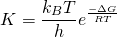Using Eyring equation, you would get the equation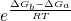.

From this result, when you estimate the reaction speed differences, you don’t need to solve the Eyring equation. Roughly saying, if the activation energy difference is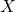kcal/mol, one reaction proceed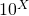faster than another. For example, let’s say the antivation energy difference is 5 kcal/mol, the main path proceed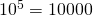times faster than the side pathway. Note that, for asymmetric synthesis, 3 kcal/mol activation energy difference gives the 99% selectivity.

#### Thermodynamic Stability

The reaction analyses using enrgy diagrams are normally a kinetics analysis issue. While discussing the stability of two compounds are thermodynamic issues.

It is said that the presence ratio will be about 99: 1 if there is a difference of 3.0 kcal/mol.

It is said that the presence ratio will be about 9: 1 if there is a difference of 1.7 kcal/mol.

It is said that the presence ratio will be about 7: 1 if there is a difference of 0.5 kcal/mol.

However, it is extremely dangerous to compare energy of such small values ​​only with DFT calculation. If it is about 0.5 kcal / mol, it will not be surprising that the magnitude of energy will change depending on the functional employed. I think that such detailed calculations in DFT should be handled as support of experimental values. Or it is better to use other calculation methods (such as complete basis set, etc.)?

* Original article was written in Japanese.

#### Related Posts

シェアする
WebmasterFollowComputational-Chemistry.com